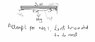# 1) A rope 5m is fastened to two hooks 4m apart on a horizontal

• verb tense
In summary, the conversation discusses problems involving ropes, poles, weights, and forces. The first problem involves a rope attached to two hooks on a ceiling and a 10kg mass. The tension in the string is to be computed, given the lengths of the rope segments. The second problem involves a 7m long pole, a boy and a man supporting it, and a 20kg weight that needs to be attached at a certain point for the man to support three times as much weight as the boy. The third problem involves a man carrying a load on a uniform pole and determining the vertical force applied by his hand and the force on his shoulder, using the rule of equilibrium. The conversation also mentions the importance of creating a diagram and

#### verb tense

1) A rope 5m is fastened to two hooks 4m apart on a horizontal ceiling. To the rope is attached a 10kg mass so that the segment of the rope are 3.0m and 2.0m.Compute the tension in the string.
2) A uniform pole 7m long weighing 10kg is supported by a boy 2m from one end and a man 3m from the other end. At what point must 20kg weight be attached so that the man would support thrice as much weight as the buy
3)A man carries a load of mass 2.6kg from one end of a uniform pole 100cm which has a mass 0.4kg the pole rests on his shoulder at a point 60cm from the load he holds it at the other hand what vertical force must be applied by his hand and what is the force on his shoulder.

Rule of equilibrium under parallel complanor force
Total downward forces = total upward forces
total anticlockwise moment= total clockwise moment
moment= force x perpendicular distance to line of action.

My attempt

#### Attachments

•Physics.jpg
13.3 KB · Views: 925

You have to imagine that you are hanging the mass as directed in the problem. Try to do that and you get the correct diagram.

OK but what's the formula for computing tension?

It all starts from the diagram according to the way I know how to do it.